Question

The Earth, which has an equatorial radius of 6380 km, makes one revolution on its axis every 23.93 hours. What is the tangential speed of Fayetteville, NC, whose latitude is 35o N

1.ThanhThu

The tangential speed of Fayetteville, NC is 1372.2 km/h.

Explanation:

To find the tangential speed at the latitude 35 °N, first, we need to calculate the radius at that latitude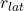: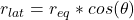Where: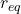: is the equatorial radius = 6380 km

θ = 35 °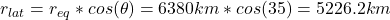Now, the tangential speed is: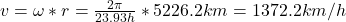Therefore, the tangential speed of Fayetteville, NC is 1372.2 km/h.

I hope it helps you!